## LetsPlayMaths.Com

WELCOME TO THE WORLD OF MATHEMATICS

# Class 2 Division

Basics of Division

2-Digits Division

3-Digits Division

Division With Remainder

Word Problem

Division Test

Division Worksheet

## Basics of Division

We can say division is a process by which equal amount of distribution can be done among a group and it is denoted as ‘÷’. Let’s have a look at some examples.

Example 1. 25 chocolates are there, it must be distributed equally among 5 boys. How many chocolates each boy should get?Solution. No. of chocolates each boy will get = Total number of chocolates ÷ Number of boys
= 25 ÷ 5
We must find out in 5 tables where 25 comes i.e. 5 X 5 = 25
That means 25 ÷ 5 = 5
Each boy should get 5 chocolates.

From the above example we conclude that division can be done using tables.

## 2-Digits Division

Lets’ know another way of dividing numbers.

Example 1. 72 ÷ 4

Solution.
Step 1.

7 tens ÷ 4 = 1 ten

Write 1 ten above 7.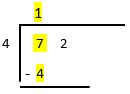Step 2. Subtract 4 from 7.

7 tens – 4 tens = 3 tensStep 3.
Bring down 2 from ones place.

3 tens + 2 ones = 32Step 4.

Divide 32 by 4. In other words, we must find out in 4’s table, where 32 comes.

4 X 8 = 32

32 ÷ 4 = 8

8 goes to the ones place. Subtract 32 from 32.

32 – 32 = 0

So, 72 ÷ 4 = 18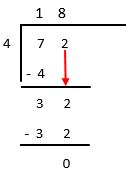Here 72 is known as dividend, 4 is known as divisor and 18 is known as quotient.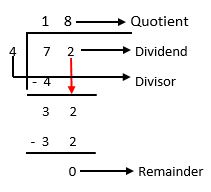## 3-Digits Division

It is similar to the 2-digit division. Let’s have a look at some examples.
Example 1. 456 ÷ 3

Solution.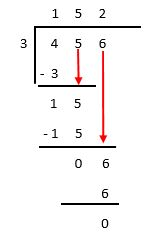Step 1.

4 hundreds ÷ 3 = 1 hundred

Write 1 hundred above 4.

Step 2.

Subtract 3 from 4.

4 hundreds – 3 hundreds = 1 hundreds

Step 3.

Bring down 5 from tens place.

1 hundreds + 5 tens = 10 tens + 5 tens = 15 tens

Divide 15 by 3. In other words, we must find out in 3’s table, where 15 comes.

3 X 5 = 15

15 ÷ 3 = 5

5 goes to the tens place of quotient. Subtract 15 from 15 i.e. 15 – 15 = 0

Step 4.

Bring down 6 from ones place of dividend.

6 ÷ 3 = 2

2 goes to the ones place of quotient.

So, 456 ÷ 3 = 152

Example 2. 675 ÷ 5

Solution.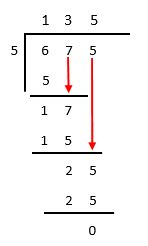## Division With Remainder

Let’s assume we have 6 chocolates and we have to divide it among 5 children equally. If we give one chocolate to each child, then 5 chocolates will be over, and 1 chocolate will remain with us. Let’s go through some examples given below.

Example 1. 75 ÷ 4

Solution.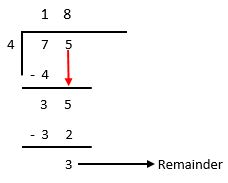Here 75 is dividend, 4 is divisor, 18 is quotient and 3 is remainder.

Example 2. 93 ÷ 5

Solution.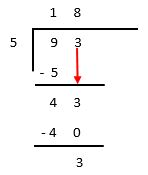Here 93 is dividend, 5 is divisor, 18 is quotient and 3 is remainder.

## Word Problem

In our day to day life division is used to solve different problems. Let’s have a look at some examples.

Example 1. Divide 65 chocolates among 5 girls. How many chocolates each girl will get?Solution.Total number of chocolates = 65
Number of girls = 5
Each girl will get = Total number of chocolates ÷ Number of girls
= 65 ÷ 5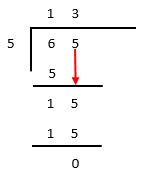Each girl will get 13 chocolates.

## Division Test

Division Test - 1

Division Test - 2

## Class-2 Division Worksheet

Division Worksheet - 1

Division Worksheet - 2

Division Worksheet - 3